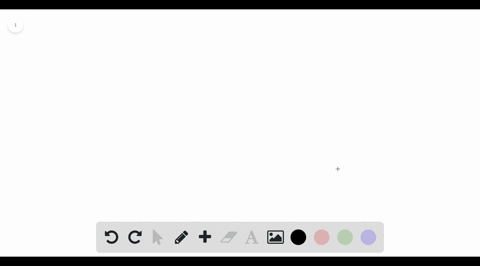Black Friday is Here! Start Your Numerade Subscription for 50% Off!Join Today### Refer to the figure and find the volume generated…

00:44Problem 18

# Find the volume of the solid obtained by rotating the region bounded by the given curves about the specified line. Sketch the region, the solid, and a typical disk or washer.$$y=x, y=0, x=2, x=4 ; \quad \text { about } x=1$$

## Discussion

You must be signed in to discuss.

## Video Transcript

in order to evaluate the integral of first thing we can do is we can draw this. Okay, Now we know our volume is gonna be pi times the integral from zero to cause those of the bounds. They've specified that in the problem. Then the top three square demise. The bottom one squared. Do you? Why now? We're not done with the integral we have toe. Add the 2nd 1 pi times from 2 to 4. Again bounds. They've given us top three squared minus or I mighta swarm. That's an expression squared. And then do you? Why this simplifies to pulling pie out? Because it's a constant as we've established. Okay, now we're at the point. We can take the derivative. We know the driven out of this in this context. We're taking that into role of us because this is the derivative of the integral. Essentially are the original function. So we have ate pie. Why? From 0 to 2. And the reason why is because we're we're adding on the variable onto a pie because we know eight pies, the driven it pie wise, We just had the very bomb. Which is gonna be Why do the same thing at the vory blam used the power rule and Christy exploded by one divide by the new exponents. Plug in and consult it like terms to get your final answer.